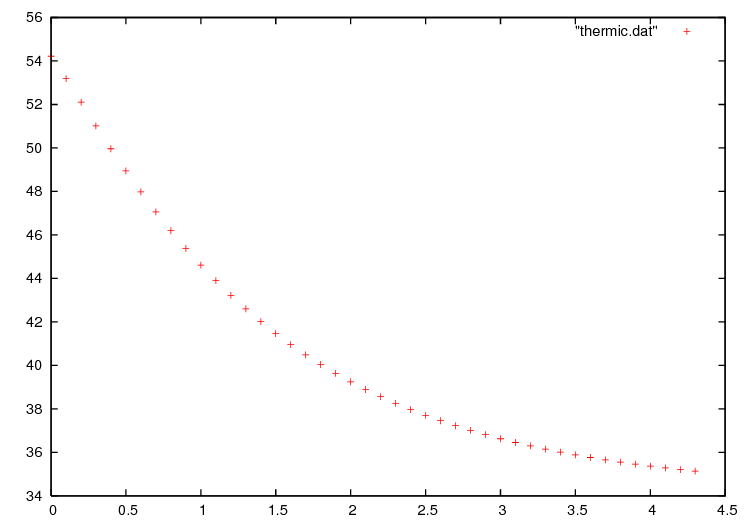FreeFEM Documentation on GitHub

stars - forks

# Thermal Conduction

Summary : Here we shall learn how to deal with a time dependent parabolic problem. We shall also show how to treat an axisymmetric problem and show also how to deal with a nonlinear problem

How air cools a plate

We seek the temperature distribution in a plate $$(0,Lx)\times(0,Ly)\times(0,Lz)$$ of rectangular cross section $$\Omega=(0,6)\times(0,1)$$; the plate is surrounded by air at temperature $$u_e$$ and initially at temperature $$u=u_0+\frac x L u_1$$. In the plane perpendicular to the plate at $$z=Lz/2$$, the temperature varies little with the coordinate $$z$$; as a first approximation the problem is 2D.

We must solve the temperature equation in $$\Omega$$ in a time interval (0,T).

$\begin{split}\begin{array}{rcl} \partial_t u -\nabla\cdot(\kappa\nabla u) &= 0 & \hbox{ in } \Omega\times(0,T)\\ u(x,y,0) &= u_0+x u_1 &\\ \kappa\frac{\partial u}{\partial \boldsymbol{n}} +\alpha(u-u_e) &= 0 & \hbox{ on } \Gamma\times(0,T) \end{array}\end{split}$

Here the diffusion $$\kappa$$ will take two values, one below the middle horizontal line and ten times less above, so as to simulate a thermostat.

The term $$\alpha(u-u_e)$$ accounts for the loss of temperature by convection in air. Mathematically this boundary condition is of Fourier (or Robin, or mixed) type.

The variational formulation is in $$L^2(0,T;H^1(\Omega))$$; in loose terms and after applying an implicit Euler finite difference approximation in time; we shall seek $$u^n(x,y)$$ satisfying for all $$w\in H^1(\Omega)$$:

$\int_\Omega(\frac{u^n-u^{n-1}}{\delta t} w + \kappa\nabla u^n\nabla w) +\int_\Gamma\alpha(u^n-u_ue)w=0$
 1// Parameters
2func u0 = 10. + 90.*x/6.;
3func k = 1.8*(y<0.5) + 0.2;
4real ue = 25.;
5real alpha=0.25;
6real T=5.;
7real dt=0.1 ;
8
9// Mesh
10mesh Th = square(30, 5, [6.*x,y]);
11
12// Fespace
13fespace Vh(Th, P1);
14Vh u=u0, v, uold;
15
16// Problem
17problem thermic(u, v)
18    = int2d(Th)(
19          u*v/dt
20        + k*(
21              dx(u) * dx(v)
22            + dy(u) * dy(v)
23        )
24    )
25    + int1d(Th, 1, 3)(
26          alpha*u*v
27    )
28    - int1d(Th, 1, 3)(
29          alpha*ue*v
30    )
31    - int2d(Th)(
32          uold*v/dt
33    )
34    + on(2, 4, u=u0)
35    ;
36
37// Time iterations
38ofstream ff("thermic.dat");
39for(real t = 0; t < T; t += dt){
40    uold = u; //equivalent to u^{n-1} = u^n
41    thermic; //here the thermic problem is solved
42    ff << u(3., 0.5) << endl;
43    plot(u);
44}


Note

We must separate by hand the bilinear part from the linear one.

Note

The way we store the temperature at point (3, 0.5) for all times in file thermic.dat. Should a one dimensional plot be required (you can use gnuplot tools), the same procedure can be used. For instance to print $$x\mapsto \frac{\partial u}{\partial y}(x,0.9)$$ one would do:

1for(int i = 0; i < 20; i++)
2   cout << dy(u)(6.0*i/20.0,0.9) << endl;


Results are shown on Fig. 13 and Fig. 14.Fig. 13 Temperature at $$t=4.9$$.Fig. 14 Decay of temperature versus time at $$x=3, y=0.5$$

Thermal conduction

## Axisymmetry: 3D Rod with circular section

Let us now deal with a cylindrical rod instead of a flat plate. For simplicity we take $$\kappa=1$$.

In cylindrical coordinates, the Laplace operator becomes ($$r$$ is the distance to the axis, $$z$$ is the distance along the axis, $$\theta$$ polar angle in a fixed plane perpendicular to the axis):

$\Delta u = {1\over r}\partial _r(r\partial _r u) + {1\over r^2}\partial ^2_{\theta\theta} u + \partial ^2_{z z}.$

Symmetry implies that we loose the dependence with respect to $$\theta$$; so the domain $$\Omega$$ is again a rectangle $$]0,R[\times]0,|[$$ . We take the convention of numbering of the edges as in square() (1 for the bottom horizontal …); the problem is now:

$\begin{split}\begin{array}{rcl} r\partial_t u-\partial _r(r\partial _r u) - \partial _z(r\partial _z u) &= 0 &\hbox{ in } \Omega\\ u(t=0) &= u_0 + \frac z{L_z} (u_1-u)&\\ u|_{\Gamma_4} &= u_0&\\ u|_{\Gamma_2} &= u_1&\\ \alpha(u-u_e) + {\partial u\over \partial\boldsymbol{n}} |_{\Gamma_1\cup\Gamma_3} &= 0& \end{array}\end{split}$

Note that the PDE has been multiplied by $$r$$.

After discretization in time with an implicit scheme, with time steps dt, in the FreeFEM syntax $$r$$ becomes $$x$$ and $$z$$ becomes $$y$$ and the problem is:

 1problem thermaxi(u, v)
2    = int2d(Th)(
3          (u*v/dt + dx(u)*dx(v) + dy(u)*dy(v))*x
4    )
5    + int1d(Th, 3)(
6          alpha*x*u*v
7    )
8    - int1d(Th, 3)(
9          alpha*x*ue*v
10    )
11    - int2d(Th)(
12          uold*v*x/dt
13    )
14    + on(2, 4, u=u0);


Note

The bilinear form degenerates at $$x=0$$. Still one can prove existence and uniqueness for $$u$$ and because of this degeneracy no boundary conditions need to be imposed on $$\Gamma_1$$.

## A Nonlinear Problem : Radiation

Heat loss through radiation is a loss proportional to the absolute temperature to the fourth power (Stefan’s Law). This adds to the loss by convection and gives the following boundary condition:

$\kappa{\partial u\over \partial\boldsymbol{n}} +\alpha(u-u_e) + c[(u + 273)^4 - (u_e+273)^4] = 0$

The problem is nonlinear, and must be solved iteratively with fixed-point iteration where $$m$$ denotes the iteration index, a semi-linearization of the radiation condition gives

${\partial u^{m+1}\over \partial\boldsymbol{n}} + \alpha(u^{m+1}-u_e)+ c(u^{m+1}-u_e) (u^m+u_e +546) ((u^m + 273)^2 + (u_e+273)^2) = 0,$

because we have the identity $$a^4 - b^4 = (a-b)(a+b)(a^2+b^2)$$.

The iterative process will work with $$v=u-u_e$$.

 1...
2// Mesh
3fespace Vh(Th, P1);
4Vh vold, w, v=u0-ue, b,vp;
5
6// Problem
8    = int2d(Th)(
9          v*w/dt
10        + k*(dx(v) * dx(w) + dy(v) * dy(w))
11    )
12    + int1d(Th, 1, 3)(
13          b*v*w
14    )
15    - int2d(Th)(
16          vold*w/dt
17    )
18    + on(2, 4, v=u0-ue)
19    ;
20
21verbosity=0; // to remove spurious FREEfem print
22for (real t=0;t<T;t+=dt){
23  vold[] = v[];// just copy DoF's, faster than interpolation pv=v;
24  for (int m = 0; m < 5; m++) {
25    vp[]=v[];// save previous state of commute error
26    b = alpha + rad * (v + 2*uek) * ((v+uek)^2 + uek^2);